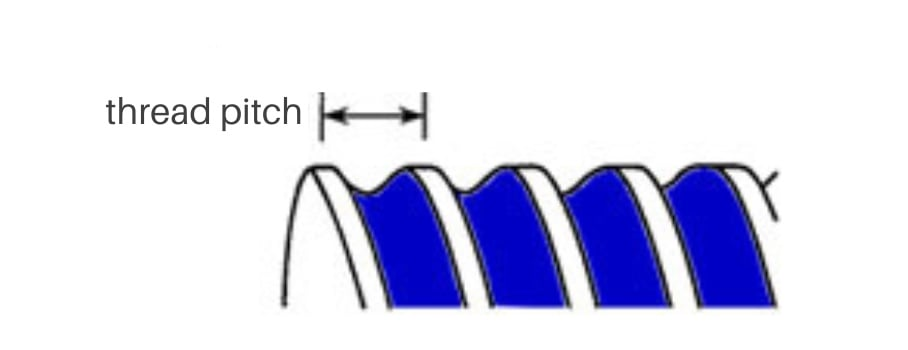Thread pitch is the distance between the same point on adjacent threads of a screw and can be referred to as TPI or threads per inch. A pitch gauge can be used to check thread pitch giving you an approximate measurement based on already determined thread standards. For a precise measurement you will need to use a caliper to measure the distance between the crests of two threads.

You can also calculate thread pitch by measuring the entire length of the thread of a screw and counting the total number of threads. You can then divide the thread length by total number of threads to calculate the thread pitch.

TP = L / n

 TP = Thread Pitch L   = Length n   = Number of threads

Calculate the TPI by dividing the number of thread (n) by the total length of the thread (L).

TPI = n / L